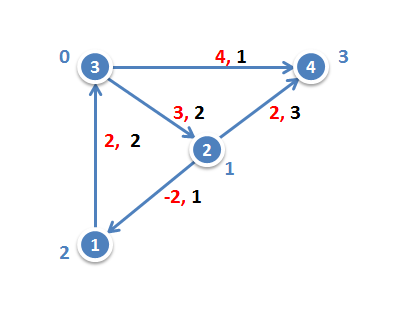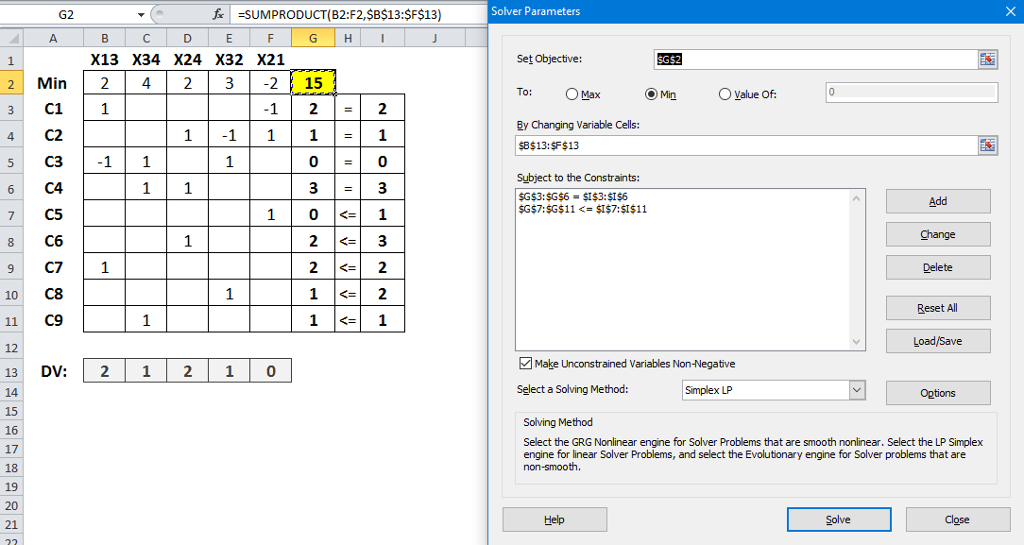# Solved: Consider the following linear program: Min 2X_13 + 4X_34 + 2X_24 + 3 X_32 – 2x_21

Consider the following linear program: Min 2X_13 + 4X_34 + 2X_24 + 3 X_32 – 2x_21 subject to X_32 – x_21 = 2 X_24 + X_21 – X_32 = 1 X_32 + X_34 – X_13 = 0 X_24 + X_34 = 3 X_21 lessthanorequalto 1 X_24 lessthanorequalto 3 X_13 lessthanorequalto 2 X_32 lessthanorequalto 2 X_34 lessthanorequalto 1 X_13, X_24, X_32, X_21, X_34 greaterthanorequalto 0 a) By drawing the appropriate network, show that this linear program can be interpreted as representing the objective and flow constraints for a min-cost flow mode a) b) Use the min-cost augmenting flow algorithm to solve your model from part (a). You may omit the details of your shortest path calculations, but otherwise show all work.

a) Network diagram showing the objective and flow constraints is as follows

In the below network diagram, cost is shown with Red numbers on the arrow. Flow constraint (capacity) is shown with Black numbers. Netflow constraint on each node is shown with Blue numbers next to each node.

Don't use plagiarized sources. Get Your Custom Essay on
Solved: Consider the following linear program: Min 2X_13 + 4X_34 + 2X_24 + 3 X_32 – 2x_21
GET AN ESSAY WRITTEN FOR YOU FROM AS LOW AS \$13/PAGEb) Solution of the problem is as followsFormulae used in the above model, G2 =SUMPRODUCT(B2:F2,\$B\$13:\$F\$13)   copy to G2:G11

X13 = 2, X34 = 1, X24 = 2, X32 = 1, X21 = 0

Objective = 15